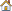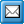﻿ Recalculate
QPR Knowledge Base 2017.1

RecalculateDeveloper's Guide > QPR API > The Object Model > QPR Metrics > SCModel Commands:

RecalculateRecalculateRevision history:

Introduced in QPR 7.2.1

Recalculate all elements for given periodId. If PeriodId is 0, then all periods are recalculated. If PeriodId is 0, then Date parameter can be used to limit the time period which is to be recalculated.

Synopsis:

SCModel.Recalculate(PeriodId, Date)

Parameters:

PeriodId: Integer. If a valid period id is given, then only that period and all its child periods are calculated. In that case the Date parameter is ignored. If this is 0, then the Date parameter is used to determine which periods are calculated. However, if Date is empty and this is 0, all periods are calculated.

Date: Date string. If this parameter is provided, then all periods that end before the specified date are included. In addition, the date needs to be provided in a format that is compatible with the regional settings of the user account QPR Metrics was launched with. This parameter is ignored in case the PeriodId parameter is provided.

Required Rights:

Return Values:

Below are listed the return values that this function can return:

RV_SUCCESS

RV_MODEL_NOT_OPEN

RV_NO_RIGHTS

RV_PERIOD_NOT_FOUND

RV_INVALID_PARAMETER

RV_UNKNOWN_ERROR

Example Procedure:

iRet = SCModel.Recalculate(0,"") 'recalculate all